# 雾星

Mistystar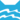河族 (Q630)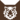虎族 (Q645)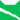雷族 (Q627)[临时][避难]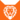狮族 (Q646)河族 学徒河族 武士河族 猫后河族 武士雷族 武士[临时]河族 副族长
（继任自石毛 (Q3084)河族 副族长河族 族长
（继任自豹星 (Q2491)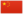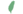小雾 Mistykit  小霧
 雾爪非官 Mistypaw  霧掌非官
 雾脚 Mistyfoot  霧足
 雾星 Mistystar  霧星

Q3214：河族母猫，原雷族猫，自《呼唤野性》登场的角色

## 细节

### 外貌

• 她是一只身形矫健、:278皮毛光滑:147厚实、:54:310蓝色眼睛:54的淡蓝灰色母猫，:20章有着粉色的鼻子:467和羽毛似的尾巴。:55
• 她生前身形孱弱，:4皮毛黯淡稀疏，口鼻泛灰。:19
• 她的外貌和母亲蓝星极为相似，:265:171-172而哥哥石毛则被描述为像是她的分身。:198

### 趣闻

• 她具有雷族血统（来自蓝星:465）。
• 在同窝手足中，她排行老二。:40章:2
• 她是目前全系列中已知最长寿的猫（大约16岁）。:466
• 由于她和黑星之间存在弑之仇，她拒绝与后者的族群结盟。:4章

## 登场书目

• 呼唤野性 (Q57)[对话提及][首次登场🐱]
• 疑云重重 (Q60)[配角]
• 风起云涌 (Q65)[配角]
• 险路惊魂 (Q66)[配角]
• 力挽狂澜 (Q67)[配角]
• 午夜追踪 (Q139)[配角]
• 新月危机 (Q140)[配角]
• 重现家园 (Q141)[配角]
• 星光指路 (Q142)[配角]
• 黄昏战争 (Q143)[配角]
• 日落和平 (Q144)[配角]
• 预视力量 (Q152)[配角]
• 暗河汹涌 (Q153)[配角]
• 驱逐之战 (Q154)[对话提及]
• 天蚀遮月 (Q155)[配角]
• 暗夜长影 (Q156)[猫物闲角]
• 拂晓之光 (Q157)[猫物闲角]
• 第四学徒 (Q158)[配角]
• 战声渐近 (Q159)[配角]
• 暗夜密语 (Q160)[配角]
• 月光印记 (Q161)[配角]
• 武士归来 (Q162)[配角]
• 群星之战 (Q163)[配角]
• 学徒探索 (Q170)[配角]
• 雷影交加 (Q171)[配角]
• 天空破碎 (Q172)[配角]
• 极夜无光 (Q173)[配角]
• 烈焰焚河 (Q174)[配角]
• 风暴来袭 (Q175)[配角]
• 迷失群星 (Q3563)[配角]
• 静默冰融 (Q3579)[配角]
• 叠影重障 (Q3624)[配角]
• 暗由心生 (Q4183)[配角]
• 无星之地 (Q4385)[配角]
• 迷雾明光 (Q4621)[配角]
• 河翻浪涌 (Q4643)[配角][死亡时刻✝]
• 天穹将倾 (Q4799)[配角]
• 火星的探索 (Q176)[配角]
• 蓝星的预言 (Q177)[配角]
• 钩星的承诺 (Q179)[配角]
• 黑莓星的风暴 (Q182)[配角]
• 虎心的阴影 (Q185)[对话提及]
• 鸦羽的拷问 (Q186)[配角]
• 松鼠飞的希冀 (Q3561)[配角]
• 灰条的誓言 (Q4057)[猫物闲角]
• 豹星的荣耀 (Q4426)[配角]
• 一星的告解 (Q4826)[猫物闲角]
• 冬青叶的故事 (Q187)[猫物闲角]
• 雾星的征兆 (Q188)[主人公][主要角色][视角人物]
• 虎掌的愤怒 (Q190)[猫物闲角]
• 叶池的祈愿 (Q191)[猫物闲角]
• 鸽翅的沉默 (Q192)[配角]
• 枫荫的报复 (Q193)[间接提及]
• 斑叶的衷肠 (Q196)[配角]
• 红尾的恩债 (Q3565)[间接提及]
• 褐皮的族群 (Q3566)[猫物闲角]
• 蛾翅的秘密 (Q3628)[配角]
• 黛西的至亲 (Q3629)[对话提及]
• 斑毛的叛变 (Q4337)[对话提及]
• 黑脚的清算 (Q4336)[对话提及]
• 河族阴云 (Q4311)[配角]
• 变革之风 (Q4411)[配角]
• 武士失踪 (Q207)[配角]
• 返回族群 (Q212)[配角]
• 族群的秘密 (Q199)[追溯修订]
• 族群的猫 (Q200)[配角]
• 族群的战争 (Q202)[配角]
• 终极指南 (Q204)[配角]
• 族群决定 (Q4580)[配角]
• 超越守则：光灵的恻隐之心 (Q4321)[配角]

更多资料：雾星/周边

## 参考文献

这篇文章基于CC BY-SA 3.0许可使用了猫武士维基（英语）Mistystar一文中的部分内容。